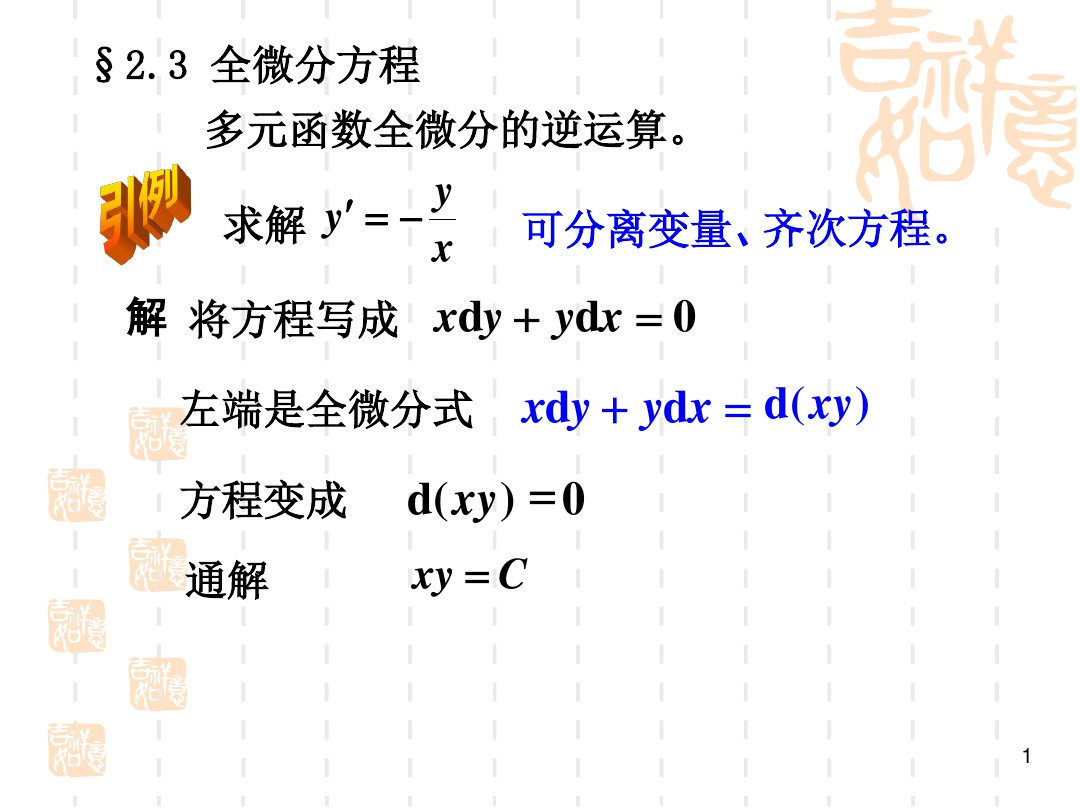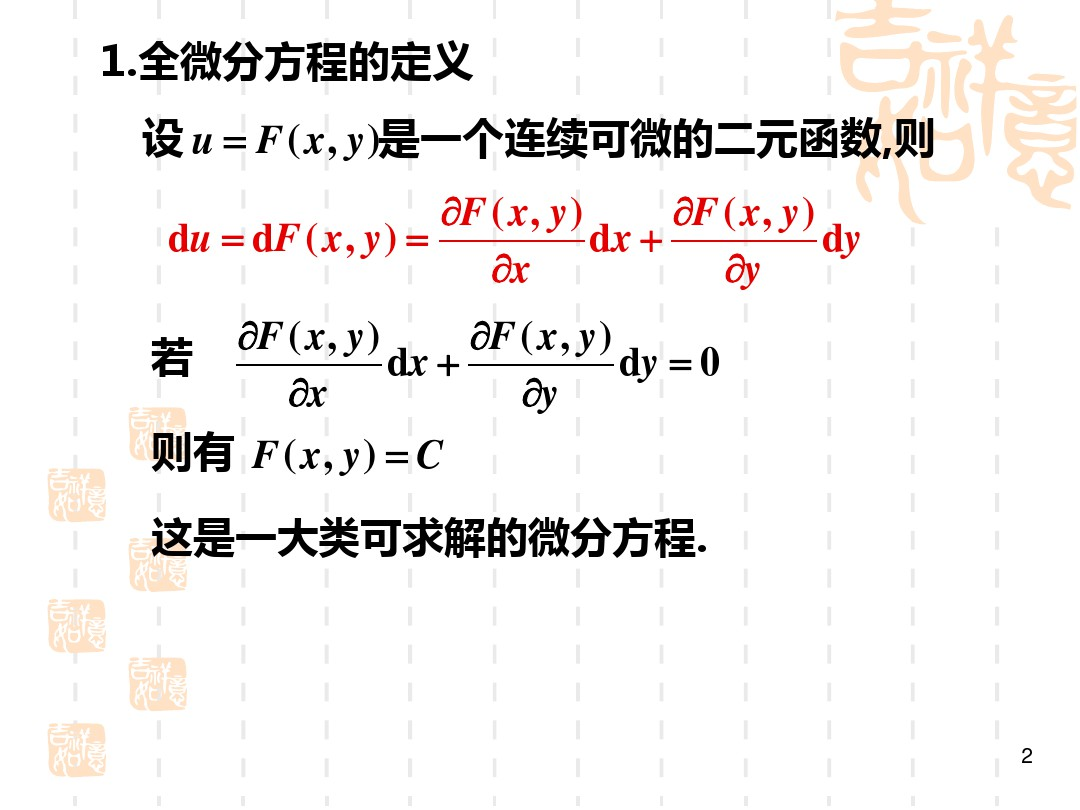# 为什么d（xy）=dxy+dyx

388 2023-05-23 16:19

d(xy)=xdy+ydx,here x ,y are both functions of t,i.e.x=x(t),y=y(t).
d(xy)/dt=(xy)'=x'y+xy'=ydx/dt+xdy/dt,multiply dt in both sides and it will lead to d(xy)=xdy+ydx.·